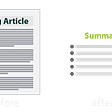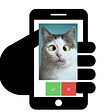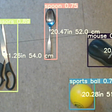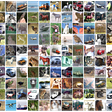# From YOLO annotation to using the weights with darknet_ros

This is a step by step explanation on:

1. How to annotate images for YOLO processing
2. How to train YOLO V3 TINY
3. How to test the weights, asap
4. How to use the weights with darknet_ros

In practice, I used these steps to train object recognition for drones. I am using Ubuntu 16.

# How to annotate images for YOLO processing

I assume you have a video of object you want to recognize, make sure you have the object recorded under different light conditions and different angles, to make sure YOLO can recognize it well.

First you need to extract frames from the video, you can use ffmpeg for that:

`ffmpeg -i myfile.mp4 extracted_frames%04d.jpg -hide_banner`

Donwload the YOLO Annotation tool, extract it, and put the extracted frames under the folder :

`Yolo-Annotation-Tool-New-/Images`

Make sure to edit the file “classes.txt”, with the classes you want to recognize. Classes are the “types” of objects you want to recognize, e.g. window, human, etc.

Make sure to put one class per line.

To start annotating, open a terminal and run:

`cd Yolo-Annotation-Tool-New-/python main.py`

A GUI should open showing your frames, you can start clicking away on the frames.

For every frame you draw boxes, a file is being created under the “Labels” folder. After you finish with every frame, make sure to put frames and labels on the same folder (you can copy them both to a new folder).

OK, now edit “process.py” with the path to your folder (labels+images). This will create train.txt and test.txt for your files.

# How to train YOLO V3 TINY

• train.txt
• test.txt
• obj.names
• obj.data

Now we have to edit the .cfg of a specific YOLO version, I will be demonstrating with YOLO v3 tiny, please take a look at the comments:

`[net]# Testing#batch=1         ##### <<<< uncomment these two lines to train,#subdivisions=1  ##### <<<< uncomment these two lines to train# Trainingbatch=32         ##### <<<< comment these two lines to test,subdivisions=8   ##### <<<< comment these two lines to testwidth=416height=416channels=3momentum=0.9decay=0.0005angle=0saturation = 1.5exposure = 1.5hue=.1learning_rate=0.001burn_in=1000max_batches = 500200policy=stepssteps=400000,450000scales=.1,.1[convolutional]batch_normalize=1filters=16size=3stride=1pad=1activation=leaky[maxpool]size=2stride=2[convolutional]batch_normalize=1filters=32size=3stride=1pad=1activation=leaky[maxpool]size=2stride=2[convolutional]batch_normalize=1filters=64size=3stride=1pad=1activation=leaky[maxpool]size=2stride=2[convolutional]batch_normalize=1filters=128size=3stride=1pad=1activation=leaky[maxpool]size=2stride=2[convolutional]batch_normalize=1filters=256size=3stride=1pad=1activation=leaky[maxpool]size=2stride=2[convolutional]batch_normalize=1filters=512size=3stride=1pad=1activation=leaky[maxpool]size=2stride=1[convolutional]batch_normalize=1filters=1024size=3stride=1pad=1activation=leaky###########[convolutional]batch_normalize=1filters=256size=1stride=1pad=1activation=leaky[convolutional]batch_normalize=1filters=512size=3stride=1pad=1activation=leaky[convolutional]size=1stride=1pad=1#filters=255filters=21   ############# 3x (num_of_classes+5)   <<<<<< edit hereactivation=linear[yolo]mask = 3,4,5anchors = 10,14,  23,27,  37,58,  81,82,  135,169,  344,319#classes=80classes=2num=6jitter=.3ignore_thresh = .7truth_thresh = 1random=1[route]layers = -4[convolutional]batch_normalize=1filters=128size=1stride=1pad=1activation=leaky[upsample]stride=2[route]layers = -1, 8[convolutional]batch_normalize=1filters=256size=3stride=1pad=1activation=leaky[convolutional]size=1stride=1pad=1#filters=255 filters=21 ############# 3x (num_of_classes+5)   <<<<< edit hereactivation=linear[yolo]mask = 0,1,2anchors = 10,14,  23,27,  37,58,  81,82,  135,169,  344,319#classes=80classes=2num=6jitter=.3ignore_thresh = .7truth_thresh = 1random=1`

After you modify the .cfg you can train:

`./darknet detector train obj.data yolov3_tiny_1class.cfg darknet19_448.conv.23`

# How to test the weights, asap

After training the desired number of batches (4000 is a good number to take a look at your weights), we can test the weights on an image (remember to comment the .cfg to test):

`./darknet detector test obj.data yolov3_tiny_1class.cfg yolov3_tiny_1class_4000.weights testingimage.jpg`

or test with a video:

`./darknet detector demo obj.data yolov3_tiny_1class.cfg yolov3_tiny_1class_4000.weights testingvideo.avi -i 0 -out_filename output.avi`

# How to use the weights with darknet_ros

catkin build it to your ros project.

`darknet_ros/darknet_ros/yolo_network_config/cfg/`

`darknet_ros/darknet_ros/yolo_network_config/weights/`

edit darknet_ros/darknet_ros/config/ros.yaml with your camera topic:

`topic: /bebop/image_raw`

create a myfile.yaml file under darknet_ros/darknet_ros/config/:

`yolo_model:config_file:    name: yolov3_tiny_1class.cfg  weight_file:    name: yolov3_tiny_1class_4000.weights  threshold:    value: 0.7  detection_classes:    names:      - window`

Create a myObjectRecognition.launch Launch file under darknet_ros/darknet_ros/launch:

`<?xml version="1.0" encoding="utf-8"?><launch>  <!-- Console launch prefix -->  <arg name="launch_prefix" default=""/><!-- Config and weights folder. -->  <arg name="yolo_weights_path"          default="\$(find darknet_ros)/yolo_network_config/weights"/>  <arg name="yolo_config_path"           default="\$(find darknet_ros)/yolo_network_config/cfg"/><!-- ROS and network parameter files -->  <arg name="ros_param_file"             default="\$(find darknet_ros)/config/ros.yaml"/>  <arg name="network_param_file"         default="\$(find darknet_ros)/config/myfile.yaml"/><!-- Load parameters -->  <rosparam command="load" ns="darknet_ros" file="\$(arg ros_param_file)"/>  <rosparam command="load" ns="darknet_ros" file="\$(arg network_param_file)"/><!-- Start darknet and ros wrapper -->  <node pkg="darknet_ros" type="darknet_ros" name="darknet_ros" output="screen" launch-prefix="\$(arg launch_prefix)">    <param name="weights_path"          value="\$(arg yolo_weights_path)" />    <param name="config_path"           value="\$(arg yolo_config_path)" />  </node><!--<node name="republish" type="republish" pkg="image_transport" output="screen"  args="compressed in:=/front_camera/image_raw raw out:=/camera/image_raw" /> --></launch>`

catkin build again your darknet_ros package and run:

`roslaunch darknet_ros myObjectRecognition.launch`

That’s it! Nice Job!

--

--

--

## More from Anna C S Medeiros

Love podcasts or audiobooks? Learn on the go with our new app.

## An Introduction to Neural Networks with Implementation from Scratch using Python## Labeling Legal Documents Using Machine Learning## Text Summarization with pretrained BART model## Scratch Neural Net Implementation — Part 3 — Complete Neural Network with Backpropagation## Machine Learning Algorithms## ML & DL Learning path## Detecting the vanishing point in one-point perspective images using Computer Vision algorithms## How to label data on your mobile phone## Estimating depth for YOLOv5 object detection bounding boxes using Intel® RealSense™ Depth Camera…## Computer Vision: Face detection using Haar Cascades## Custom DataLoader for Deep learning## Object recognition and handling overfitting for CIFAR-10 image dataset